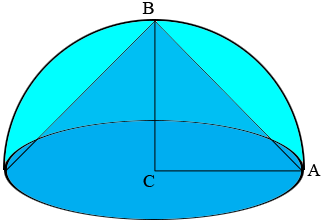SEARCH HOMEMath Central Quandaries & QueriesQuestion from anonymous, a student: A cone is inscribed in a hemisphere. the slant height of the cone is 20cm. When cut along its slant height, the cone forms a sector of a circle. find the angle of the sector, to the nearest 1 decimal place.Hi,

In my diagram$CA$ and $BC$ are both radii of the hemisphere and hence are of the same length, call it $R$ cm. Triangle $ABC$ is an isosceles right triangle with hypotenuse of length 20 cm so Pythagoras Theorem will help you find the value of $R.$ Now use the procedure in Harley's response to an earlier question to complete the solution.

PennyMath Central is supported by the University of Regina and the Imperial Oil Foundation.